# Payroll Support Document

Open as: PDF or Word Document

## 2. Calculate Payroll

Calculate Payroll

All

Department

Calculate Single Posting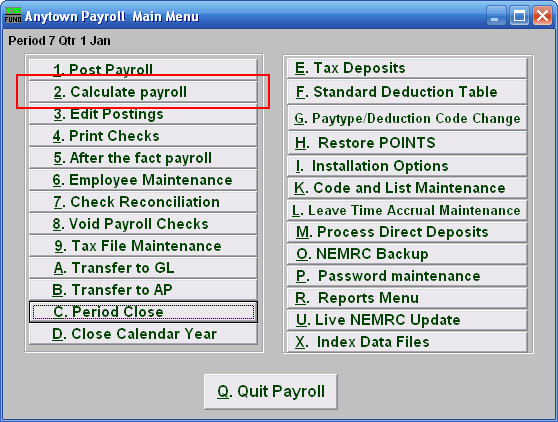Click on "2. Calculate payroll" from the Main Menu and the following window will appear:

Calculate Payroll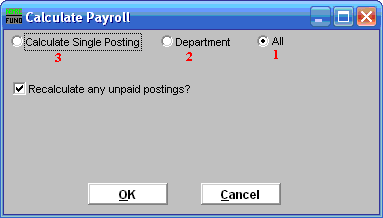1. All: Click to Calculate Payroll for all Employees.
2. Department: Click to Calculate Payroll for a Department only.
3. Calculate Single Posting: Click to Calculate Payroll for a single Employee.

Refer to the section below that relates to your choice.

All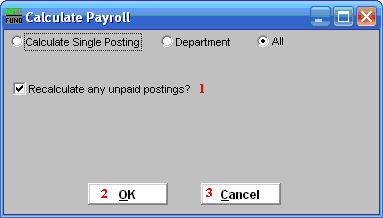1. Recalculate any unpaid postings?: Click to check the box and have the system Recalculate any Postings that have not been paid. This will remove and recalculate the taxes. This will recalculate all fringes and deductions that are based upon a percentage value.
2. OK: Click "OK" to Calculate.

Department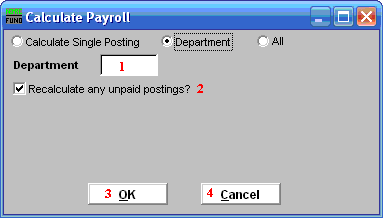1. Department: Enter the department value found in Employee Maintenance for the group to calculate.
2. Recalculate any unpaid postings?: Click to check the box and have the system Recalculate any Postings that have not been paid. This will remove and recalculate the taxes. This will recalculate all fringes and deductions that are based upon a percentage value.
3. OK: Click "OK" to Calculate.

Calculate Single Posting

If you click "Edit," the following window will appear: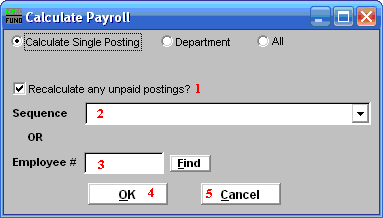1. Recalculate any unpaid postings?: Click to check the box and have the system Recalculate any Postings that have not been paid. This will remove and recalculate the taxes. This will recalculate all fringes and deductions that are based upon a percentage value.
2. Sequence: Select the sequence number assigned by the system for which to calculate.
3. Employee #: Enter the Employee number for which to calculate.
4. OK: Click "OK" to Calculate.